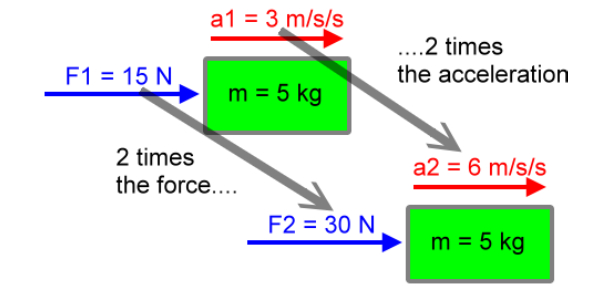# Free Body Diagrams: Calculating Net Force And Acceleration

8 Questions | Total Attempts: 993SettingsCreate your own QuizTo calculate net force: add vectors in the same direction; subtract vectors in the opposite direction. To calculate acceleration: acceleration = net force / mass Use the following abbreviations for units: newtons = N meters per second squared = m/ss Use the following for directions: right, left, up, downBack to top